# Why is energy saved in mechanical systems

The 1st law of thermodynamics is a special form of the law of conservation of energy in mechanics. It says that energies can be transformed into one another, but cannot be formed or destroyed. For the transition of a closed system from state A to B it reads: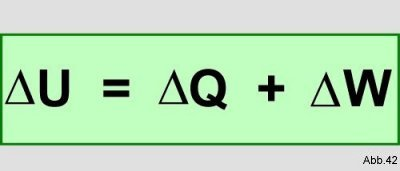In words this means: The change in the internal energy of a closed system is equal to the sum of the change in heat and the change in work.
This means that the internal energy in a closed system is constant. Work done on the system or the amount of heat added is given a positive sign and work done by the system or the amount of heat given off is given a negative sign.
The total amount of energy in a system that has passed from state A to state B is consequently the sum of the energies supplied as heat and work. This total amount of energy is the internal energy U. For their numerical value, it does not matter whether heat or work was added. It is therefore independent of the path and consequently a state variable.
If you look at an isolated system, neither heat nor work or any other energy can escape. Energy can neither be created nor destroyed. A change in an isolated system can therefore lead neither to an increase nor to a decrease in internal energy, but only to a redistribution of the amount of energy between the different forms.
In the case of closed systems, this applies if the environment of the system is included in the consideration, which would then again correspond to an isolated system as a whole. If the internal energy U changes in a closed system, the internal energy of the environment must change by the same amount, but with the opposite sign.

Explanation of the individual components:

1. The internal energy U:

Internal energy is a form of energy that is stored by the smallest particles of a substance, the atoms or molecules. This takes place in the form of motion, rotation and vibration energy.
The internal energy U is an equation of state, it only depends on its current state, but not on the way in which it was reached.

2. The heat Q:
For a long time, no precise distinction was made between the two terms warmth and temperature. In earlier centuries the term was used for both calor used.
A system can be changed with the addition of work in the same way as with the addition of heat. For example, if you stir water, it heats up. It does the same when it is brought into contact with a warmer body. Therefore it was assumed that heat is also a form of energy. One of the first scientists to distinguish between the two sizes was Joseph Black. He showed, among other things, that heat is an extensive quantity and temperature is an intensive quantity. The doctor Robert Mayer and the brewer James Prescott Joule also made numerous attempts in this direction from 1840-1850.
If you consider two systems between which there is a temperature difference, energy (in the form of heat) is transferred from the colder to the warmer until both have the same temperature. The prerequisite is that the two systems are in thermal contact with one another. In open systems, however, heat can also be transferred from one system to another by mass transfer.

3. The work W:
If a force shifts particles in their position, it does work. This work can also be done on a system. It is not, however, that work is added to the system, or that a system contains work. work is no Material. Work is a path function because its numerical value depends on the path through which it is reached.
An acting force can, for example, perform volume work on a system. A gas is considered, in this case hydrogen (H.2), which is enclosed in a glass cylinder with a movable disc, which has the area A:The gas experiences a pressure that is calculated from the weight F of the disk and its area A: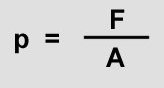(26)

The force F can be increased by placing weights on the disk, for example.
If the system is in equilibrium, the disc is at rest. In this case, the pressure of the enclosed gas is just as great as the pressure from outside: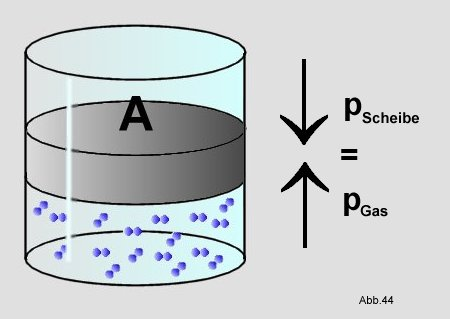If you increase the pressure from outside with a weight of 500 g, the disk moves down and compresses the hydrogen: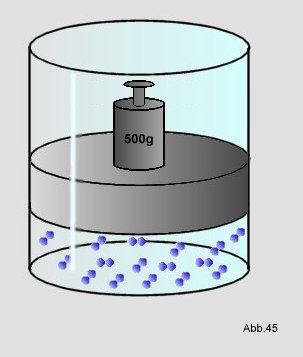This happens until there is an equilibrium again between the new higher pressure and the counter pressure of the compressed hydrogen.
The movement of the disk does work W on the hydrogen:(27)

p is the pressure and DV is the change in volume of the gas. The change in volume DV is calculated by multiplying the area A of the piston by the change in height Dh of the enclosed gas volume:(28)

Ie is determined as follows:The volume becomes smaller when the piston moves a distance Dh. It follows from this that if Dh is positive, the change in volume is negative. The work done is also positive as the gas has been compressed.

Perpetual motion machine:
A machine that produces energy out of nothing, i.e. supplies energy without being supplied in the same amount, is called a `` Perpetual motion machine of the 1st kind ".
From the statement of the 1st law it becomes clear that such a machine cannot exist. An energy gain would only have to be obtained from an energy redistribution. Thus D U should be> 0. However, this contradicts the 1st law, which says the following:Where A and B denote 2 different states and s and u are abbreviations for system or environment.
The unsuccessful efforts of numerous researchers and inventors also confirm the non-existence of such a machine.

Relationship with the enthalpy H:
If a reaction is carried out at constant pressure in such a way that only volume work can be done, then the following applies to work W (formula (27)):(27)

with p = pressure and DV = change in volume
If you put that in the 1st main clause, you get the following:(29)

converted you get: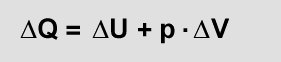(30)

In this case, Q is called enthalpy H: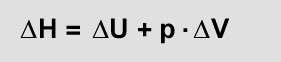(31)

The enthalpy change DH is defined as the amount of heat that is absorbed by a closed isobaric system when it undergoes a change of state at which no work other than volume work occurs. If heat is released into the environment, the enthalpy of the system decreases (DH is negative) and the reaction is exothermic. Heat absorption means that it is an endothermic reaction (DH positive). In a reaction, the sign and the numerical value of DH can almost only be ascribed to the changes in energy that are related to the closing, breaking or joining of chemical compounds.